• 4.2.5 预测分析法与预测分析表的构造
千次阅读
2020-08-07 10:16:38

## 4.2.5 预测分析法与预测分析表的构造

预测分析法也称为 LL ( 1 )分析法。这种分析法是确定的自上而下分析的另一种方法,采用这种方法进行语法分析要求描述语言的文法是 LL ( 1 )文法。
一个预测分析器由一张预测分析表(也称为 LL ( 1 )分析表)、一个先进后出分析栈和一个总控程序三部分组成,见图 4.3 。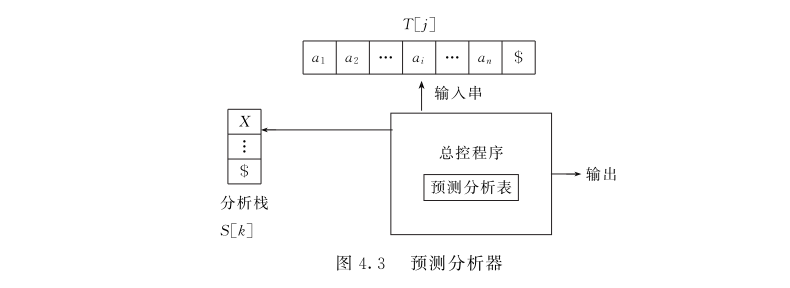图中输入缓冲区 T [j ]中存放待分析的输入符号串,它以右界符 $作为结束。分析栈S [ k ]中存放替换当前非终结符的某规则右部符号串,句子左界符“$ ”存入栈底。预测分析表是一个 M [ A ,a ]形式的矩阵,其中 A 为非终 结符, a 是终结 符或“ $”。分析表元素M [ A , a ]中的内容为一条关于 A 的规则,表明当 A 面临输入符号 a 时,当前推导所应采用的候选式,当元素的内容为“出错标志”(表中用空白格表示“出错标志”)时,则表明 A 不应该面临输入符号 a 。 预测分析器的总控程序在任何时候都是根据栈顶符号和当前输入符号 a 来决定分析器的动作,其工作过程用流程图 4.4 表示。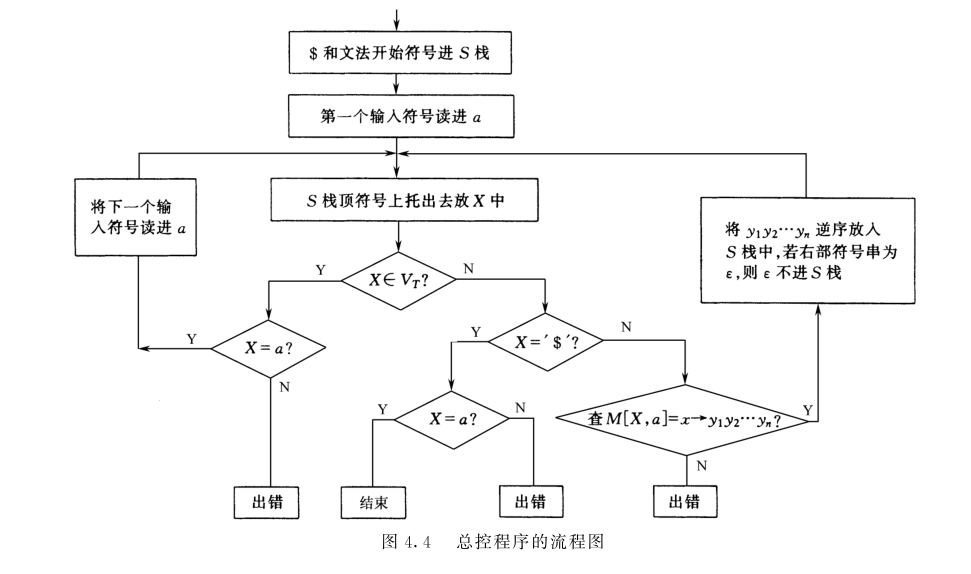预测分析器的总控程序对于不同的 LL ( 1 )文法都是相同的,而预测分析表对于不同的LL ( 1 )文法是不相同的,下面我们介绍对于任给的文法 G 构造预测分析表的算法。 输入:文法 G 。 输出:预测分析表 M 。 方法: (1 )计算文法 G 的每一非终结符的 FIRST 集和 FOLLOW 集。 ① 对每一文法符号 X ∈ ( V N ∪ V T ),计算 FIRST ( X ): a. 若 X ∈ V T ,则 FIRST ( X ) = { X }。 b. 若 X ∈ V N 且有规则 X → a …, a ∈ V T ,则 a ∈FIRST ( X )。 c. 若 X ∈ V N 且有规则 X → ε ,则 ε ∈FIRST ( X )。 d. 若有规则 X → y 1 y 2 … y n ,对于任意的 i ( 1≤ i ≤ n ),当 y 1 y 2 … y i -1 都是非终结符,且y 1 y 2 … y i -1 ⇒* ε 时,则将 FIRST ( y i )中的非 ε 元素加到 FIRST ( X )中。 特别是,若 y 1 y 2 … y n ⇒* ε,则 ε ∈FIRST ( X )。 e. 反复使用 a. ~d. 直到 FIRST 集不再增大为止。  ② 对文法中的每一个 A ∈ V N ,计算 FOLLOW ( A ): a. 对文法的开始符号 S ,则将“$ ”加到 FOLLOW ( S )中。

b. 若 A → αB β 是一条规则,则把 FIRST (β )中的非 ε 元素加到 FOLLOW (B )中。

c. 若 A → αB 或 A → αB β 是一条规则且 β ⇒* ε,则把 FOLLOW ( A )加到 FOLLOW ( B )中。

d. 反复使用 b.~c. 直到每个非终结符的 FOLLOW 集不再增大为止。


(2 )对文法的每个规则 A → α ,若 a ∈FIRST ( α ),则置 M [ A , a ] = A → α 。

(3 )若 ε ∈FIRST ( α ),对任何 b ∈FOLLOW ( A ),则置 M [ A , b ] = A → α 。

(4 )把分析表中无定义的元素标上出错标志 error (表中用空白格表示)。

【例 4.10 】设有文法 G [ S ]:

S → a |∧| ( T )
T → ST'
T' → , ST' | ε


试构造该文法的预测分析表。
分析 首先判断该文法是否 LL ( 1 )文法,在例 4.9 中已经证明该文法是 LL ( 1 )文法。
计算该文法每个非终结符的 FIRST 集和 FOLLOW 集: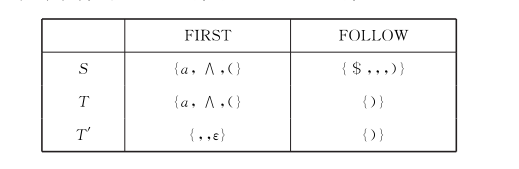对规则 S → a ,因为 FIRST (a ) = { a },所以置 M [ S , a ] = S → a 。

对规则 S →∧ ,因为 FIRST ( ∧ ) = { ∧ },所以置 M [ S , ∧ ] = S →∧

对规则 S → ( T ),因为 FIRST (( T )) = {(},所以置 M [ S ,(] = S → ( T )。

对规则 T → ST’ ,因 为 FIRST ( ST’ ) = {(,a , ∧ },所 以 置 M [ T ,(] = T → ST’ ,M [ T , a ] = T → ST’ , M [ T , ∧ ] = T → ST’ 。

对规则 T’ → ,ST’ ,因为 FIRST (, ST’ ) = {,},所以置 M [ T’ ,]。 = T’ → , ST’ 。

对规则 T’ → ε ,因为 FOLLOW ( T’ ) = {)},所以置 M [ T’ ,)] = T’ → ε 。
文法 G [ S ]的分析表见表 4.1 。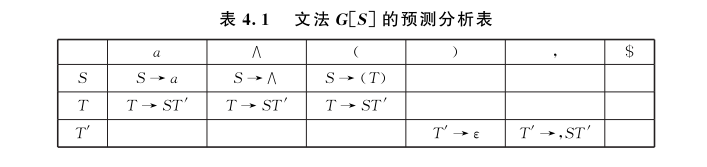对输入串(a , a ) \$ 预测分析器作出的移动如表 4.2 所示。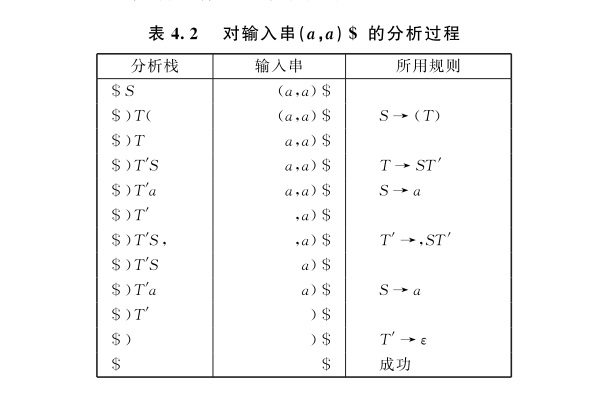可以证明,若一个文法 G 的分析表 M 不含多重定义元素,则该文法是 LL ( 1 )文法。

更多相关内容
• 根据文法编制预测分析法语法分析程序，以便对输入的符号串进行语法分析。通过编写预测分析法语法分析程序掌握预测分析法的基本原理、FIRST和FOLLOW集的计算、预测分析表的构造方法以及语法分析法主控程序的设计。

# 一、实验目的

根据文法编制预测分析法语法分析程序，以便对输入的符号串进行语法分析。通过编写预测分析法语法分析程序掌握预测分析法的基本原理、FIRST和FOLLOW集的计算、预测分析表的构造方法以及语法分析法主控程序的设计。

# 二、实验内容

对于给定的上下文无关文法，编程完成以下功能：
（1）消除左递归
（2）计算非终结符的FIRST和FOLLOW集
（3）构造预测分析表
（4）判断消除了左递归后的文法是否为LL(1)文法
（5）编写预测分析法语法分析程序，要求对输入的任意符号串进行语法分析，输出推导过程或语法分析树。

# 三、实验要求

1、 输入/输出格式。文法的输入示例参考cfg1.txt以及cfg2.txt。例如文法以以下形式给出：

E::=E + T
E::=E - T
E::=T
T::=T * F
T::=T / F
T::=F
F::=( E )
F::=id


文法只给出产生式列表，每个产生式占一行，产生式右边的文法符号串每个符号之间有一个空格，末尾没有空格。第一个产生式左边的非终结符为开始符号。如果某非终结符A的候选式为ε，则产生式表示为“A::=”，即产生式的右部为空。（文法的输入格式也可以以你觉得合适的方式定义）
输出的结果按照实验内容依次输出消除左递归后的文法，非终结符的FIRST和FOLLOW集，预测分析表、是否为LL（1）文法，以及输出测试字符串利用预测分析法进行语法分析。
2、上述要求仅为基本要求，可以在此基础上扩充。例如能够处理间接左递归，增加错误处理，输出错误信息等。进一步可以指出出错的位置。
3、编程语言不限。 本文使用C语言来实现。

# 四、实验设计方案# 五、测试方案及测试结果

输入文法消除左递归计算非终结符的FIRST集计算非终结符的FOLLOW构造预测分析表输入要进行分析的符号串输出分析过程# 结语

预测分析法语法分析器的设计的介绍就到这里啦，希望这篇文章能给予你一些帮助，感谢各位人才的：点赞、收藏和评论，我们下次见。

# 附录

以下提供测试代码
预测分析法语法分析器的设计

#include <stdio.h>
#include <stdlib.h>
#define MaxRuleNum 10
#define MaxVnNum 10
#define MaxVtNum 10
#define MaxStackDepth 20
#define MaxPLength 20
#define MaxStLength 50
#include <conio.h>

struct pRNode { //产生式右部的结构
int rCursor;//指向字符表的指针表示产生式右部字符
struct pRNode *next;//链表的组织结构
};
struct pNode {
int lCursor; //指向产生式左边字符
int rLength; //产生式右部分的长度
};

char Vn[MaxVnNum + 1]; //非终结符集
int vnNum;

char Vt[MaxVtNum + 1]; // 终结符集
int vtNum;

struct pNode P[MaxRuleNum];//产生式集
int PNum;

char buffer[MaxPLength + 1];//缓冲串集合
char ch;

char st[MaxStLength]; //要分析的符号串

struct collectNode { //定义first和follow集
int nVt;//字符
struct collectNode *next;
};

struct collectNode* first[MaxVnNum + 1]; //first 集每个元素是个指向链表的指针
struct collectNode* follow[MaxVnNum + 1]; //follow 集

int analyseTable[MaxVnNum + 1][MaxVtNum + 1 + 1];//分析表
int analyseStack[MaxStackDepth + 1]; //分析栈
int topAnalyse; /* 分析栈顶 */
void Init();/* 初始化 */
int IndexCh(char ch);
void InputVt(); /* 输入终结符 */
void InputVn();/* 输入非终结符 */
void ShowChArray(char* collect, int num);/* 输出 Vn 或 Vt 的内容 */
void InputP();/* 产生式输入 */
bool CheckP(char * st);/* 判断产生式正确性 */
void First(int U);
void AddFirst(int U, int nCh); /* 加入 first 集*/
bool HaveEmpty(int nVn);
void Follow(int V);/* 计算 follow 集*/
void AddFollow(int V, int nCh, int kind);
void ShowCollect(struct collectNode **collect);/* 输出 first 或 follow集*/
void FirstFollow();/* 计算 first 和 follow*/
void CreateAT();/* 构造预测分析表 */
void ShowAT();/* 输出分析表 */
void Identify(char *st);
void InitStack();
void ShowStack();
void Pop();
void Push(int r);
void printp();//输出所有产生式
void isdturn();//消除直接左递归
void isjturn();//消除间接左递归
void length();//更新长度

int main() {
char todo, ch;
Init();
InputVn();
InputVt();
InputP();
getchar();//吃掉换行
isjturn();
isdturn();
length();
printf(" 所得不含左递归的产生式为：\n");
printp();
FirstFollow();
printf(" 所得 first 集为： ");
ShowCollect(first);
ShowCollect(follow);
CreateAT();
ShowAT();
todo = 'y';
while ('y' == todo) {
printf("\n 是否继续进行句型分析？ (y / n):");
todo = getchar();
while ('y' != todo && 'n' != todo) {
printf("\n(y / n)? ");
todo = getchar();
}
if ('y' == todo) {
int i;
InitStack();
printf(" 请输入符号串 ( 以#结束 ) : ");
ch = getchar();
i = 0;
while ('#' != ch && i < MaxStLength) {
if (' ' != ch && '\n' != ch) {
st[i++] = ch;
}
ch = getchar();
}
if ('#' == ch && i < MaxStLength) {
st[i] = ch;
Identify(st);
}
else
printf(" 输入出错！ \n");
}
}
getchar();
}
void Init() {
int i, j;
vnNum = 0;
vtNum = 0;
PNum = 0;
for (i = 0; i <= MaxVnNum; i++)
Vn[i] = '\0';//非终初始化
for (i = 0; i <= MaxVtNum; i++)
Vt[i] = '\0';//终初始化
for (i = 0; i < MaxRuleNum; i++) {//产生式初始化
P[i].lCursor = NULL;
P[i].rLength = 0;
}
for (i = 0; i <= MaxPLength; i++)
buffer[i] = '\0';//字符集初始化
for (i = 0; i < MaxVnNum; i++) {
first[i] = NULL;//first初始化
follow[i] = NULL;//follow初始化
}
for (i = 0; i <= MaxVnNum; i++) {
for (j = 0; j <= MaxVnNum + 1; j++)
analyseTable[i][j] = -1;//分析表的初始化
}
}
int IndexCh(char ch) {
int n;
n = 0; /*is Vn?*/
while (ch != Vn[n] && '\0' != Vn[n])
n++;
if ('\0' != Vn[n])
return 100 + n;//非终的值是大于100的
n = 0; /*is Vt?*/
while (ch != Vt[n] && '\0' != Vt[n])
n++;
if ('\0' != Vt[n])
return n;
return -1;//空返回-1
}

void ShowChArray(char* collect) {
int k = 0;
while ('\0' != collect[k]) {
printf(" %c ", collect[k++]);
}
printf("\n");
}

void InputVn() {
int inErr = 1;
int n, k;
char ch;
while (inErr) {
printf(" 请输入所有的非终结符，注意： ");
printf(" 请将开始符放在第一位，并以 #号结束 :\n");
ch = ' ';
n = 0;
/* 初始化数组 */
while (n < MaxVnNum) {
Vn[n++] = '\0';
}
n = 0;
while (('#' != ch) && (n < MaxVnNum)) {
if (' ' != ch && '\n' != ch && -1 == IndexCh(ch)) {//排除空格,换行,已经输入的字符
Vn[n++] = ch;
vnNum++;
}
ch = getchar();
}
Vn[n] = '#'; /* 以"#" 标志结束用于判断长度是否合法 */
k = n;
if ('#' != ch) {
if ('#' != (ch = getchar())) {
while ('#' != (ch = getchar()))
;//清除缓冲区,一会重新输入不能在读进去了
printf("\n 符号数目超过限制！ \n");
inErr = 1;
continue;
}
}
/* 正确性确认，正确则，执行下下面，否则重新输入 */
Vn[k] = '\0';
ShowChArray(Vn);
ch = ' ';
while ('y' != ch && 'n' != ch) {
if ('\n' != ch) {
printf(" 输入正确确认 ?(y/n):");
}
scanf("%c", &ch);
}
if ('n' == ch) {
printf(" 录入错误重新输入！ \n");
inErr = 1;
}
else {
inErr = 0;
}
}
}
/* 输入终结符 */
void InputVt() {
int inErr = 1;
int n, k;
char ch;
while (inErr) {
printf("\n 请输入所有的终结符，注意： ");
printf(" 以#号结束 :\n");
ch = ' ';
n = 0;
/* 初始化数组 */
while (n < MaxVtNum) {
Vt[n++] = '\0';
}
n = 0;
while (('#' != ch) && (n < MaxVtNum)) {
if (' ' != ch && '\n' != ch && -1 == IndexCh(ch)) {
Vt[n++] = ch;
vtNum++;
}
ch = getchar();
}
Vt[n] = '#';//默认最后一个是#
k = n;
if ('#' != ch) {
if ('#' != (ch = getchar())) {
while ('#' != (ch = getchar()))
;
printf("\n 符号数目超过限制！ \n");
inErr = 1;
continue;
}
}
Vt[k] = '\0';
ShowChArray(Vt);
ch = ' ';
while ('y' != ch && 'n' != ch) {
if ('\n' != ch) {
printf(" 输入正确确认 ?(y/n):");
}
scanf("%c", &ch);
}
if ('n' == ch) {
printf(" 录入错误重新输入！ \n");
inErr = 1;
}
else {
inErr = 0;
}
}
}
/* 产生式输入 */
void InputP() {
char ch;
int i = 0, n, num;
printf(" 请输入文法产生式的个数： ");
scanf("%d", &num);
PNum = num;
getchar(); //删除回车
printf("\n 请输入文法的 %d个产生式 , 并以回车分隔每个产生式： ", num);
printf("\n");
while (i < num) {
printf(" 第%d 个： ", i);
/* 初始化 */
for (n = 0; n < MaxPLength; n++)
buffer[n] = '\0';
/* 输入产生式串 */
ch = ' ';
n = 0;
while ('\n' != (ch = getchar()) && n < MaxPLength) {
if (' ' != ch)//除去空格换行
buffer[n++] = ch;
}
buffer[n] = '\0';
if (CheckP(buffer)) {
pRNode *pt, *qt;
P[i].lCursor = IndexCh(buffer);//产生式左边赋值
pt = (pRNode*)malloc(sizeof(pRNode));//构造产生式右部
pt->rCursor = IndexCh(buffer);
pt->next = NULL;
n = 4;
while ('\0' != buffer[n]) {//构造链表
qt = (pRNode*)malloc(sizeof(pRNode));
qt->rCursor = IndexCh(buffer[n]);
qt->next = NULL;
pt->next = qt;
pt = qt;//pt跟着qt
n++;
}
P[i].rLength = n - 3;//产生式右部长度
i++;
}
else
printf(" 输入符号含非法在成分，请重新输入！ \n");
}
}
/* 判断产生式正确性 */
bool CheckP(char * st) {
int n;
if (100 > IndexCh(st))//如果第一个字符是终结符
return false;
if ('-' != st)//终结符后面不是->则报错
return false;
if ('>' != st)
return false;
for (n = 3; '\0' != st[n]; n++) {
if (-1 == IndexCh(st[n]))//输入了不存在的报错
return false;
}
return true;
}
//消除间接左递归
void isjturn()
{
for (int j = 0; j<vnNum; j++)
{
for (int i = 0; i < PNum; i++)
{
if (j == (P[i].lCursor - 100) && P[i].rHead->rCursor >= 100 && P[i].rHead->rCursor<P[i].lCursor)
{
for (int k = 0; k<i; k++)
{
{
pRNode * qt = (pRNode*)malloc(sizeof(pRNode));
while (p != NULL)
{

pRNode * pt = (pRNode*)malloc(sizeof(pRNode));
pt->rCursor = p->rCursor;
qt->next = pt;
qt = pt;
p = p->next;
}
}

}

}
}
}
}
//消除直接左递归
void isdturn()
{

int flag2 = 0;

struct collectNode *pa;//作为a的数组
struct collectNode *pb;
for (int i = 0; i<10; i++)
{
pa[i] = pb[i] = NULL;
}
int n = 0, m = 0;
pRNode * p = NULL;
for (int j = 0; j<vnNum; j++)
{
int flag1 = 0;
for (int i = 0; i < PNum; i++)
{

if (j == P[i].lCursor - 100 && j == P[i].rHead->rCursor - 100)
{
flag1 = 1; break;
}
}
if (flag1 == 1)
{
for (int i = 0; i < PNum; i++)
{
if (j == (P[i].lCursor - 100) && j == (P[i].rHead->rCursor - 100))
{
n++;
P[i].lCursor = vnNum + 100;
pRNode* pt = (pRNode*)malloc(sizeof(pRNode));//构造产生式右部
pt->rCursor = vnNum + 100;
pt->next = NULL;
while (p->next != NULL) p = p->next;
p->next = pt;
}
if (j == (P[i].lCursor - 100) && j != (P[i].rHead->rCursor - 100) && -1 != P[i].rHead->rCursor)
{
pRNode* pt = (pRNode*)malloc(sizeof(pRNode));//构造产生式右部
pt->rCursor = vnNum + 100;
pt->next = NULL;
while (p->next != NULL) p = p->next;
p->next = pt;
}
}
P[PNum++].lCursor = vnNum + 100;
pRNode* pt = (pRNode*)malloc(sizeof(pRNode));//构造产生式右部
pt->rCursor = -1; pt->next = NULL;
Vn[vnNum++] = Vn[j] + 32;
}

}
}

void First(int U) {
int i, j;
for (i = 0; i < PNum; i++) {//对于每一个产生式
if (P[i].lCursor == U) {//如果产生式的左边是该非
struct pRNode* pt;
j = 0;
while (j < P[i].rLength) {
if (100 > pt->rCursor) {//如果是终结符
break;
}
else {
if (NULL == first[pt->rCursor - 100]) {//非终结符如果它first集合空
First(pt->rCursor);//求他的first集合
}
if (!HaveEmpty(pt->rCursor)) {
break;
}
else {
pt = pt->next;//如果该非终的first有空找下一个
}
}
j++;
}
if (j >= P[i].rLength) // 当产生式右部都能推出空时 才把空加进去
}
}
}

/* 加入 first 集*/
void AddFirst(int U, int nCh) {//把nch加到终结符U的first集
struct collectNode *pt, *qt;
int ch; /* 用于处理 Vn*/
pt = NULL;
qt = NULL;
if (nCh < 100) {//对于终结符
pt = first[U - 100];//找到它的first集合的链表
while (NULL != pt) {
if (pt->nVt == nCh)
break;
else {
qt = pt;
pt = pt->next;//qt记录前一,pt一直到空
}
}
if (NULL == pt) {
pt = (struct collectNode *)malloc(sizeof(struct collectNode));
pt->nVt = nCh;
pt->next = NULL;
if (NULL == first[U - 100]) {//如果本来就没有,则直接将他作为头结点
first[U - 100] = pt;
}
else {
qt->next = pt; //新加入到first集合的字符连到链表上
}
pt = pt->next;
}
}
else {//终结符
pt = first[nCh - 100];
while (NULL != pt) {
ch = pt->nVt;
if (-1 != ch) {
}
pt = pt->next;
}
}
}
bool HaveEmpty(int nVn) {//非终且能推出空则true
if (nVn < 100)
return false;
struct collectNode *pt;
pt = first[nVn - 100];
while (NULL != pt) {
if (-1 == pt->nVt)
return true;
pt = pt->next;
}
return false;
}
void Follow(int V) {
int i;
struct pRNode *pt;
if (100 == V) // 当为字符是初始字符的时候
for (i = 0; i < PNum; i++) {
while (NULL != pt && pt->rCursor != V)
pt = pt->next;//产生式的有部找V
if (NULL != pt) {//找到了
pt = pt->next;
if (NULL == pt) {//是最后一个字符
if (NULL == follow[P[i].lCursor - 100] && P[i].lCursor != V) {//把左侧非V且有follow的非的集合加进去(含空)
Follow(P[i].lCursor);
}
}
else {
while (NULL != pt && HaveEmpty(pt->rCursor)) {//因为没有判断fellow集合是否不变的机制,所以需要一直看到头
pt = pt->next;//每一个非终的first
}
if (NULL == pt) {//如果后面没有了
if (NULL == follow[P[i].lCursor - 100] && P[i].lCursor != V) {
Follow(P[i].lCursor);//那么说明这个非终是最后一个,那么把产生式左边的fello加进去
}
}
else {
}
}
}
}
}
void AddFollow(int V, int nCh, int kind) {
struct collectNode *pt, *qt;
int ch;
pt = NULL;
qt = NULL;
if (nCh < 100) { /* 为终结符时直接加入链表尾部 */
pt = follow[V - 100];
while (NULL != pt) {
if (pt->nVt == nCh)
break;
else {
qt = pt;
pt = pt->next;
}
}
if (NULL == pt) {
pt = (struct collectNode *)malloc(sizeof(struct collectNode));
pt->nVt = nCh;
pt->next = NULL;
if (NULL == follow[V - 100]) {
follow[V - 100] = pt;
}
else {
qt->next = pt;
}
pt = pt->next;
}
}
else {//非终结符的时候
if (0 == kind) {//直接把follow加进去
pt = follow[nCh - 100];
while (NULL != pt) {
ch = pt->nVt;
pt = pt->next;
}
}
else {//把不含空的first加进去
pt = first[nCh - 100];
while (NULL != pt) {
ch = pt->nVt;
if (-1 != ch) {
}
pt = pt->next;
}
}
}
}
/* 输出 first 或 follow 集*/
void ShowCollect(struct collectNode **collect) {
int i;
struct collectNode *pt;
i = 0;
while (NULL != collect[i]) {
pt = collect[i];
printf("\n%c:\t", Vn[i]);
while (NULL != pt) {
if (-1 != pt->nVt) {
printf(" %c", Vt[pt->nVt]);
}
else
printf(" #");//把空转化成"#"
pt = pt->next;
}
i++;
}
printf("\n");
}
/* 计算 first 和 follow*/
void FirstFollow() {
int i;
i = 0;
while ('\0' != Vn[i]) {
if (NULL == first[i])
First(100 + i);//非终结符对应的序号在100外
i++;
}
i = 0;
while ('\0' != Vn[i]) {
if (NULL == follow[i])
Follow(100 + i);
i++;
}
}
/* 构造预测分析表 */
void CreateAT() {
int i;
struct pRNode *pt;
struct collectNode *ct;
for (i = 0; i < PNum; i++) {//对每个产生式
while (NULL != pt && HaveEmpty(pt->rCursor)) {//非终且能推出空
ct = first[pt->rCursor - 100];
while (NULL != ct) {
if (-1 != ct->nVt)
analyseTable[P[i].lCursor - 100][ct->nVt] = i;//产生式左侧遇到它的右侧第一个非终的first集合
ct = ct->next;
}
pt = pt->next;
}
if (NULL == pt) {//后面都可以推出空
ct = follow[P[i].lCursor - 100];
while (NULL != ct) {
if (-1 != ct->nVt)
analyseTable[P[i].lCursor - 100][ct->nVt] = i;
else//follow空其实就是遇到了#
analyseTable[P[i].lCursor - 100][vtNum] = i;//最后一列是#
ct = ct->next;
}
}
else {//最后一个不能推出空
if (100 <= pt->rCursor) { /* 不含空的非终结符 */
ct = first[pt->rCursor - 100];
while (NULL != ct) {
analyseTable[P[i].lCursor - 100][ct->nVt] = i;
ct = ct->next;
}
}
else { /* 非空的终结符或者空 */
if (-1 == pt->rCursor) {//相当于是推出了空
ct = follow[P[i].lCursor - 100];
while (NULL != ct) {
if (-1 != ct->nVt)
//if(analyseTable[P[i].lCursor - 100][ct->nVt] ==-1 )
analyseTable[P[i].lCursor - 100][ct->nVt] = i;
else /* 当含有 # 号时 */
analyseTable[P[i].lCursor - 100][vtNum] = i;
ct = ct->next;
}
}
else { /* 为终结符 */
analyseTable[P[i].lCursor - 100][pt->rCursor] = i;
}
}
}
}
}
/* 输出分析表 */
void ShowAT() {

int i, j;
printf(" 构造预测分析表如下： \n");
printf("\t|\t");
for (i = 0; i < vtNum; i++) {
printf("%c\t", Vt[i]);//行头是所有终
}
printf("#\t\n");//#单独输出
printf("- - -\t|- - -\t");
for (i = 0; i <= vtNum; i++)
printf("- - -\t");
printf("\n");
for (i = 0; i < vnNum; i++) {
printf("%c\t|\t", Vn[i]);//列头是终结符
for (j = 0; j <= vtNum; j++) {
if (-1 != analyseTable[i][j])
printf("R(%d)\t", analyseTable[i][j]);
else
printf("     \t");
}
printf("\n");
}
}

void Identify(char *st) {//构造栈
int current, step, r; /*r 表使用的产生式的序号 */
printf("\n%s 的分析过程： \n", st);
printf(" 步骤 \t 分析符号栈 \t 当前指示字符 \t 使用产生式序号 \n");
step = 0;//第几步
current = 0;//输入串指针
printf("%d\t", step);
ShowStack();//栈你初始就有#和开始符号
printf("\t\t%c\t\t- -\n", st[current]);
while ('#' != st[current]) {//当输入串剩下#结束
if (100 > analyseStack[topAnalyse]) {
if (analyseStack[topAnalyse] == IndexCh(st[current])) {
Pop();//终结符匹配,则出栈
current++;
step++;
printf("%d\t", step);
ShowStack();
printf("\t\t%c\t\t 出栈、后移 \n", st[current]);
}
else {
printf("%c-%c 不匹配！ ", analyseStack[topAnalyse], st[current]);
printf(" 此串不是此文法的句子！ \n");
return;
}
}
else { /* 当为非终结符时 */
r = analyseTable[analyseStack[topAnalyse] -
100][IndexCh(st[current])];
if (-1 != r) {
Push(r);//push里有pop
step++;
printf("%d\t", step);
ShowStack();
printf("\t\t%c\t\t%d\n", st[current], r);
}
else {
printf(" 此串不是此文法的句子！ \n");
return;
}
}

}
if ('#' == st[current]) {
if (0 == topAnalyse && '#' == st[current]) {
step++;
printf("%d\t", step);
ShowStack();
printf("\t\t%c\t\t 分析成功！ \n", st[current]);
printf("%s 是给定文法的句子！ \n", st);
}
else {
while (topAnalyse > 0) {
if (100 > analyseStack[topAnalyse]) {//终结符肯定不是
printf(" 此串不是此文法的句子！ \n");
return;
}
else {//虽然输入串此时是#当站内是非终是,只有遇到推出空才行
r = analyseTable[analyseStack[topAnalyse] - 100][vtNum];
if (-1 != r) {
Push(r);
step++;
printf("%d\t", step);
ShowStack();
if (0 == topAnalyse && '#' == st[current]) {//可能有多个符号条件的非
printf("\t\t%c\t\t 分析成功！ \n", st[current]);
printf("%s 是给定文法的句子！ \n", st);
}
else
printf("\t\t%c\t\t%d\n", st[current], r);
}
else {
printf(" 此串不是此文法的句子！ \n");
return;
}
}
}
}
}
}
/* 初始化栈及符号串 */
void InitStack() {
int i;
/* 分析栈的初始化 */
for (i = 0; i < MaxStLength; i++)
st[i] = '\0';
analyseStack = -1; /*#(-1) 入栈 */
analyseStack = 100; /* 初始符入栈 */
topAnalyse = 1;//栈顶指针,指向栈顶元素
}
/* 显示符号栈中内容 */
void ShowStack() {
int i;
for (i = 0; i <= topAnalyse; i++) {
if (100 <= analyseStack[i])
printf("%c", Vn[analyseStack[i] - 100]);
else {
if (-1 != analyseStack[i])
printf("%c", Vt[analyseStack[i]]);
else
printf("#");
}
}
}
/* 栈顶出栈 */
void Pop() {
topAnalyse--;
}
void Push(int r) {
int i;
struct pRNode *pt;
Pop();//
if (-1 == pt->rCursor)
return;//产生式右部是空不进栈
topAnalyse += P[r].rLength;
for (i = 0; i < P[r].rLength; i++) {
analyseStack[topAnalyse - i] = pt->rCursor;/* 逆序入栈 */
pt = pt->next;
}
}
//输出所有产生式
void printp()
{
for (int i = 0; i < PNum; i++)
{
putchar(Vn[P[i].lCursor - 100]);
printf("->");
while (pt != NULL)
{
if (pt->rCursor >= 100)
putchar(Vn[pt->rCursor - 100]);
else putchar(Vt[pt->rCursor]);
pt = pt->next;
}
printf("\n");
}
}

void length()//更新有部产生式的长度
{
for (int i = 0; i < PNum; i++)
{
int count = 0;
while (pt != NULL)
{
pt = pt->next;
count++;
}
P[i].rLength = count;
}

}



文法的输入示例

cfg1.txt

E::=E + T
E::=E - T
E::=T
T::=T * F
T::=T / F
T::=F
F::=( E )
F::=id

E->E + T
E->E - T
E->T
T->T * F
T->T / F
T->F
F->( E )
F->i


cfg2.txt

S::=( L )
S::=a
L::=L , S
L::=S

展开全文c语言
• 语法分析：自上而下分析 目录语法分析：自上而下分析知识背景计算海明校验码步骤一：计算校验码位数步骤二：确定校验组步骤三：计算校验码的值得出海明校验码利用海明校验码校验数据其他总结 知识背景 百度百科： ...

# 知识背景

百度百科 “语法分析是编译过程的一个逻辑阶段。语法分析的任务是在词法分析的基础上将单词序列组合成各类语法短语，如“程序”，“语句”，“表达式”等等.语法分析程序判断源程序在结构上是否正确.源程序的结构由上下文无关文法描述.语法分析程序可以用YACC等工具自动生成。”

语法分析在编译中也是一个比较很重要的环节，通常情况下语法分析可以分为自上而下分析和自下而上分析。
本文主要介绍自上而下分析（从文法的开始符号出发）的大致框架和细节，并且附上我写的一些代码供大家讨论。首先我看了挺多的关于自上而下的分析，感觉这里一个老师的解释比较清晰，供大家参考 链接。接下来的内容，根据这里老师讲的一个大概框架，写一些我自己的理解，然后分析一下我的代码。

要想进行自上而下分析，我们可以用LL(1)分析法来分析，而这个分析法主要有两种具体实现：递归分析法预测分析法，有可能其他地方不是这么叫的，但是分析的方法大同小异。
在讨论两种方法前，首先要讨论一下一些预备知识：

Q1:什么是自上而下分析法？自上而下分析的前提是什么？

百度百科 “自上而下分析法是从文法开始符号开始，不断进行推导，直到推导所得的符号串与输入串相同为止。”

简单来解释这句话：

我们有一个既定的文法，和一个需要分析的符号串。接下来我们从文法的开始符号出发，反复地使用文法规定的一些产生式匹配符号串的每一个符号，直到所得的符号串和待分析的符号串相同，则为分析成功，反之匹配不成功。举个例子说明：
G(E)：
E→aF
F→b|c

待分析的输入串：ab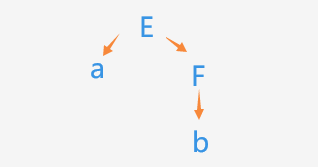从文法开始符号E出发，E→aF，a匹配成功后，指针指向F，找非终结符F的产生式合适的候选式 b匹配，于是匹配成功。

想要对一个文法进行自上而下的分析，要消除文法的二义性，消除左递归，提取左公共因子，计算FIRST集合和FOLLOW集合，判断文法是否为LL(1)型文法，一个文法经过这些步骤，并且是LL(1)文法，则可以用LL(1)分析法的两个具体实现去分析。

Q2:什么是分析过程中的回溯问题？
从上面的例子我们可以看出，在我们碰到非终结符的时候要把非终结符用它对应的产生式的右部来代替，但是一个非终结符往往不止一个候选式（比如上面那个例子的F，F→b|c就有b和c两个选项），这个时候就会出现一个问题，如果我们选择候选式来替代非终结符的时候不能准确判断，这一次的替代是否能够正确推导出匹配的结果，一旦选择的候选式不能推导成功，就要返回上一步，换一个候选式进行推导，这就是“回溯”。例如上面那个例子，我们在拓展F的时候选择了c，会发现匹配失败，然后再返回上一步，选择另一个候选式b，才匹配成功。

Q3:如何解决回溯的问题？
实际上，含有回溯的分析过程并非不可取，只是会浪费很多的资源和时间，因此我们要想办法消除回溯，也就是争取让每一次选择候选式都选择正确的那一个。这就涉及到了下文要讲的FIRST、FOLLOW集合了。

Q4:什么是文法中含有左递归？
左递归分为直接左递归和间接左递归

• 直接左递归
举一个小例子G(E)：E→Ea
在这个文法中虽然只有一个产生式，但是对这个产生式进行构造语法树的时候会发现：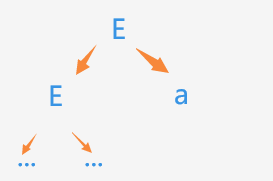这个树会无限向下扩展，无法匹配结束。
• 间接左递归
举一个小例子G(E):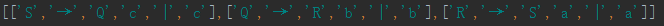这个文法推导三次后会发现又回到了文法的开始符号又一次出现了，因此又进入循环，无法结束匹配，这就是间接左递归。

Q5:如何解决文法含有左递归的问题？
对于直接左递归，我们通常把它转换成右递归很好理解，举个小例子：
G(E)：E→Ea |b
对于这个含有直接左递归的文法，将它转换成右递归的具体做法就是引入一个新的非终结符，通常我们用当前非终结符加 ’ 来表示，将文法改为
G(E)：
E→bE’
E’ →aE’|ε
可以很容易地证明这两个文法是等价的。

对于间接左递归，通常把非终结符带入来产生直接左递归，例如：
G(E):把S右部中的Q用Q的产生式代替，再把Q中的R用R的产生式代替，删除无关产生式后，就能得到一个含有直接左递归的文法：
G(E): S→Sabc|abc|bc|c
然后再将这个产生式按照直接左递归的处理方法进行消除左递归处理。

更一般地，想要让程序自动地消除左递归，具体的做法如下：
1.把文法的所有非终结符进行排序S = [‘A’,‘B’,…]
2.做一个嵌套循环：
其中S[k]为排在S[j]之后的非终结符,bunch_i为非终结符和终结符组成的串

for j in range(len(s)):
for k in range(j):
原产生式中:S[j]→S[k]bunch_1的S[k]用其对应的产生式代替
S[k]→bunch_2|bunch_3|...
推出：S[j]→bunch_2 bunch_1|bunch_3 bunch_1|...
如此，做完循环后该文法若有间接左递归，就将其转换成直接左递归了
消除直接左递归，具体做法见上文
循环结束后删除无用产生式


下面截取一些我写的消除左递归的代码进行讨论，完整源码下载请点击 下载

获取每一条产生式的所有候选式：

            temp_split = [] # 将每一个产生式分割以便求的长度来进行分类讨论
# 获取这一条产生式的所有'|'的索引值
temp_or = []
temp_push = []
for q in range(len(lst[j])):
if lst[j][q] == '|':
temp_or.append(q)
# 获取这一条产生式'→'的索引值
temp_get = lst[j].index('→')
# 转换成列表方便组合
temp_push.append(temp_get)
# 合并查找索引列表
temp_search =  temp_push + temp_or
# 把一个产生式以'→'和'|'为分隔符分割，将分割后的数据存储在嵌套列表temp_split里
for m in range(len(temp_search)):
if len(temp_search) == 1:
temp_split_1 = [lst[j][temp_search[m] + 1:][:]]
temp_split = temp_split_1[:]
else:
if m == (len(temp_search) - 1):
temp_split.append(lst[j][temp_search[m] + 1:])
else:
temp_split.append(lst[j][temp_search[m] + 1:temp_search[m + 1]])


间接左递归转换成直接左递归：

            change = [s[j],"→"]
# change = []
print("ts:",temp_split)
for n in temp_split:
if "'" in n:
n[n.index("'") - 1] += "'"
n.remove("'")
try:
if n == s[k]:
k_push = lst[k][:]
temp_z = []
for z in range(len(k_push)):
if k_push[z] == '|':
temp_z.append(z)
for x in temp_z:
for c in n[1:]:
k_push.insert(x,c)
for c in n[1:]:
if len(c) != 1:
k_push.extend(c)
else:
k_push.append(c)
change.extend(k_push)
change.append("|")
else:
if len(n) == 1:
change.append("".join(n))
else:
change.extend(n)
change.append("|")
except:
print("mark")
lst[j] = change
print("change1:",lst[j])


Q6:什么是提取左公因子？
类似数学上的提取公因式，把形如
E→bunch_1bunch_2|bunch_1bunch_3|…|bunch_1bunch_n|其他开头不是bunch_1的候选式
的产生式改写成 :
E→bunch_1E’|其他开头不是bunch_1的候选式
E’→bunch_2|bunch_3|…|bunch_n

的形式

有话说： 通常情况下，提取左公因子要反复进行，直到所有非终结符的FIRST集合两两不相交。

提取左公因子的代码比较简单，完整代码见 链接

Q7:什么是文法符号的FIRST集，非终结符的FOLLOW集，任意串的FIRST集？
构造这些FIRST集和FOLLOW集的主要目的是为了消除回溯，也就是选择候选式的时候能够更加准确，当然，这些集合在别的地方也有用处。关于Q7，参见我的另外一篇文章：FIRST / FOLLOW集合

Q8:什么是LL(1)型文法？如何判断文法是否为LL(1)型？
若一个文法G为LL(1)文法，则应满足以下条件：
1.文法不含有左递归
2.文法中每一个非终结符产生式中每一个候选式的FIRST集两两不相交（可以反复提取左公因子来接近这个条件）
3.如果文法中的每一个非终结符的FIRST集若含有ε，则每一个候选式的FIRST集和该非终结符的FOLLOW集不相交

下面截取一些我写的判断文法是否为LL(1)文法的代码进行讨论，完整源码下载请点击 下载
下面代码中temp_split为当前产生式的所有候选式的列表，例如E→a|b|c，temp_split = [[‘a’],[‘b’],[‘c’]]

		for n in range(len(temp_split) - 1):
for q in range(1,len(temp_split)):
if len(set(get_first_bunch(temp_split[n],temp_lst)) & set(get_first_bunch(temp_split[q],temp_lst))) != 0:
print("error.")
flag = 0
break
for n in range(len(temp_split)):
if 'ε' in get_first_bunch(temp_split[n],temp_lst):
if len(set(get_first_bunch(temp_split[n],temp_lst)) & set(get_follow(temp_lst)["".join(j[:j.index("→")])])) != 0 :
print("error.")
flag = 0
break


Q9:什么是LL(1)分析法？
使用LL(1)分析法的前提见Q1
LL(1)分析法：
假设当前要匹配的输入串符号为a

for i in 每一个候选式:
if a in FIRST(i):
选择候选式
if a not in 每一个候选式的FIRST集
if a in FOLLOW(当前非终结符) and ε in FIRST(每一个候选式):
选择ε
else:
error();


下面就LL(1)分析法的两种具体实现方式进行分析。

# 递归下降分析法

递归下降分析法，顾名思义就是使用递归的思想去分析，具体的步骤：

对于一个文法G，对其每一个非终结符U构造一个递归过程，一般的，以非终结符的名字来命名这个子过程。所有子程序构造完成后，对指定文法，运行文法开始符号对应的子程序，返回匹配结果。

下面分析每一步的具体操作。

## 内容一：根据文法生成子程序

在实践这一部分的代码的时候，我想了挺久，没有思路，因为要构造指定文法的递归子程序，但在编码的时候，我们并不知道用户要输入的文法结构是怎么样的，所以这就产生了这么一个问题：如何用函数构造函数？实际上，如果我们能知道文法结构，那么就可以硬编码来生成对应的子程序。但是为了程序的良好拓展性，最后我用了这一方法：
1.create_function.py运行后， 用户输入一个指定文法
2.程序读取该文法的所有非终结符并且创建一个字典以所有非终结符作为key，其值为该非终结符对应的子程序的名称（一般和非终结符同名）
3.根据子程序的一般结构，定义每一个子程序不同的部分（比如子程序名称）
4.用python的文件操作，往新文件function.py中写入子程序的内容，包括必要的import，全局变量和每一个子程序，其中每一个子程序用一个循环来写入代码。

有话说： 在编写写入新文件的代码的时候需要记得’\n’，或者按行写入。

那么子程序的一般结构是什么呢？
例如：对于一个产生式E→AC|BD|ε，word为当前读入的符号

def E(...):
if word in FIRST(AC):
A(...)
C(...)
elif word in FIRST(BD):
B(...)
D(...)
elif word in FOLLOW(A):
不做其他操作
else:
error()


具体代码举例分析，完整代码见 链接

引入需要用到的其他文件里的方法，这里我引用了我之前写的求FIRST / FOLLOW集合的方法，这里的bunch就是要匹配的输入串，是在我们create这个子程序的时候由用户输入的，q为指针，用来指向当前匹配的符号。IP当初我用来检查每一个环节输出是否正确。

import syntactic_parser_demo

bunch = "q#"
q = 0
IP = bunch


获取当前匹配字符到word中，判断匹配是否结束

 	global q
global IP
try:
word = bunch[q]
print("当前处理符号串符号：",word)
IP = word
except:
IP = "!"
return
if word == '#':
return


获取当前产生式的所有候选式，存在temp_split列表中,例如E→a|b|c，temp_split = [[‘a’],[‘b’],[‘c’]]

	temp_or = []
temp_push = []
for k in range(len(parser)):
if parser[k] == '|':
temp_or.append(k)
temp_get = parser.index('→')
temp_push.append(temp_get)
temp_search = temp_push + temp_or
temp_split = []
for m in range(len(temp_search)):
if len(temp_search) == 1:
temp_split = [parser[temp_search[m] + 1:]]
else:
if m == (len(temp_search) - 1):
temp_split.append(parser[temp_search[m] + 1:])
else:
temp_split.append(parser[temp_search[m] + 1:temp_search[m + 1]])


判断是否需要递归调用其他子程序。
在这里我用eval(n[temp_n.index(j)])(i,lst_origin)来递归调用其他子程序，但实际上eval在某些场合需要谨慎使用。python中exec也可以执行一个字符串中的表达式例如：exec ("print('1')")

有话说： exec的返回值始终为 None 。

	finish = 0
for n in temp_split:
if word == "".join(n):
q += 1
temp_n = n[:]
if "'" in temp_n:
temp_n[temp_n.index("'") - 1] += "'"
temp_n.remove("'")
if "'" in n:
n[n.index("'") - 1] += '1'
n.remove("'")
if word in syntactic_parser_demo.get_first_bunch(temp_n,lst_origin):
finish = 1
for j in temp_n:
for i in lst_origin:
if "".join(i[:i.index('→')]) == j:
eval(n[temp_n.index(j)])(i,lst_origin)
break
break


获取FOLLOW集，判断第二个条件，判断第三个条件

    dict_origin = syntactic_parser_demo.get_follow(lst_origin)
lst_follow_use = dict_origin["".join(parser[:parser.index('→')])]
if word in lst_follow_use:
finish = 1
if finish == 0:
error()

return IP



下面取一部分写入子程序的一些核心代码，完整代码见 链接

这里的写入部分我是将要写入的所有代码整合成一个字符串，再写入文件。写入的内容，先按照某一个子程序的结构，写出一个子程序的例子，再根据这个例子来写入代码。

# 创建相应的子程序并写入文件
back = open("function.py","a",encoding = "UTF-8")
back.seek(0)
back.truncate()

back.write("import syntactic_parser_demo\n\n")
back.write("bunch = " + "\"" + bunch + "\"" + "\nq = 0\nIP = bunch\n\n")
for j in lst:
if "'" in j[:2]:
temp = "".join(j) + "1"
else:
temp = "".join(j)
content = "def " + temp + "(word,parse,lst_origin):\n"\
+ "    global q\n" + "    global IP\n" + "    try:\n"\
+ "        word = bunch[q]\n" + "        print(\"当前处理符号串符号：\",word)\n"\
+ "        IP = word\n" + "    except:\n" + "        IP = \"!\"\n"\
+ "        return\n" + "    if word == '#':\n" + "        return\n"\
+ "    temp_or = []\n" + "    temp_push = []\n" + "    for k in range(len(parse)):\n"\
+ "        if parse[k] == '|':\n" + "            temp_or.append(k)\n" + "    temp_get = parse.index('→')\n"\
+ "    temp_push.append(temp_get)\n" + "    temp_search = temp_push + temp_or\n" + "    temp_split = []\n"\
+ "    for m in range(len(temp_search)):\n" + "        if len(temp_search) == 1:\n"\
+ "            temp_split = [parse[temp_search[m] + 1:]]\n" + "        else:\n"\
+ "            if m == (len(temp_search) - 1):\n"\
+ "                temp_split.append(parse[temp_search[m] + 1:])\n" + "            else:\n"\
+ "                temp_split.append(parse[temp_search[m] + 1:temp_search[m + 1]])\n"\
+ "    finish = 0\n" + "    for n in temp_split:\n" + "        if word == \"\".join(n):\n"\
+ "            q += 1\n" + "        temp_n = n[:]\n"\
+ "        if \"\'\" in temp_n:\n" + "            temp_n[temp_n.index(\"'\") - 1] += \"\'\"\n"\
+ "            temp_n.remove(\"\'\")\n" + "" + "        if \"\'\" in n:\n"\
+ "            n[n.index(\"\'\") - 1] += '1'\n"\
+ "            n.remove(\"\'\")\n"\
+ "        if word in syntactic_parser_demo.get_first_bunch(temp_n,lst_origin):\n"\
+ "            finish = 1\n"\
+ "            for j in temp_n:\n" + "                for i in lst_origin:\n"\
+ "                    if \"\".join(i[:i.index('→')]) == j:\n"\
+ "                        eval(n[temp_n.index(j)])(word,i,lst_origin)\n"\
+ "                        break\n" + "            break\n"\
+ "    dict_origin = syntactic_parser_demo.get_follow(lst_origin)\n"\
+ "    lst_follow_use = dict_origin[\"\".join(parse[:parse.index('→')])]\n"\
+ "    if word in lst_follow_use:\n" + "        finish = 1\n"\
+ "    if finish == 0:\n" + "        error()\n\n"\
+ "    return IP\n\n"
back.write(content)
back.write("def error():\n    print('error.')\n    return \n")
back.close()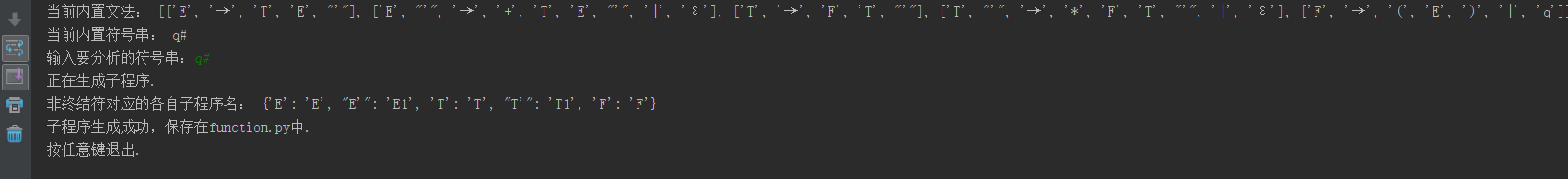## 内容二：调用文法开始符号所对应的子程序

接下来就是递归下降分析法的主程序部分，这部分比较简单，这里import function不能省略因为下面用的是eval来调用文法开始符号对应的子程序。

import function

def error():
print("error.")
return

if __name__ == "__main__":
lst_demo = [["E","→","T","E","'"],["E","'","→","+","T","E","'","|","ε"],["T","→","F","T","'"],
["T","'","→","*","F","T","'","|","ε"],["F","→","(","E",")","|","q"]]
print("当前内置文法：",lst_demo)

try:
ip = eval("function." + lst_demo)(lst_demo,lst_demo)
print("ip:",ip)
if ip == '#':
print("匹配文法成功")
else:
print("Error.")
except:
print("找不到子程序，即当前没有找到function.py或其为空文本，请先运行create_function.py创建.")
n = input("按任意键退出.")

n = input("按任意键退出.")


至此，递归下降分析法就完成了，可以少量修改代码让用户输入文法和输入串来达到更好的交互性。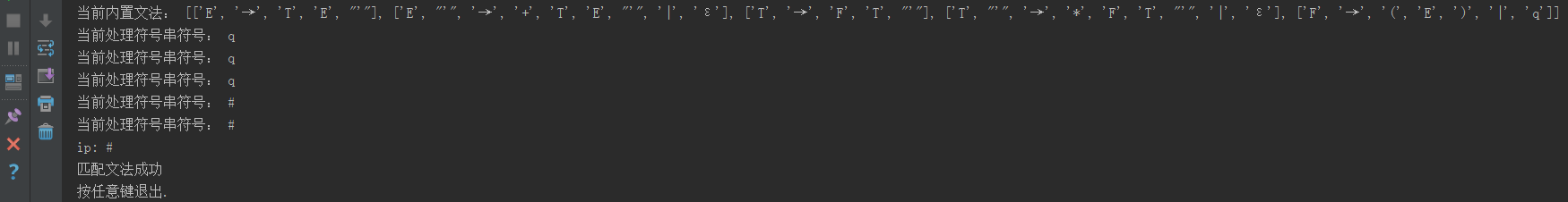# 预测分析法

预测分析法是LL(1)分析法的另一种实现方法，它不需要构造每一个子程序，而是通过一张表来关联非终结符和终结符，这张表就是预测分析表，预测分析表可以说是预测分析法的核心部分。

## 内容一：构造预测分析表

关于预测分析表的构造，参见我之前的一篇文章 构造预测分析表

## 内容二：预测分析法主程序

这里我们用一个栈来存放过程数据，主要步骤如下：
1.获取栈顶的元素A，获取输入串目前指针指向的元素a
2.若A = ‘#’ ,a = ‘#’ 则匹配成功
3.若A = a 但是A和a不为’#’，则pop栈顶元素，输入串指针+1
4.若A为非终结符，这查询预测分析表，把由A和a确定的产生式的右部从右往左依次压入到栈中，若右部是ε，那就不做操作
5.查找预测分析表得到预设的出错字符则调用error()

下面截取一些我写的代码进行讨论，完整代码见 链接

主程序的一些预备工作，这里我用列表来代替实现栈的一些功能

# 预测分析程序

import lexical_analysis_table

# 形参lst为所有产生式，bunch为待分析符号串
def control(lst,bunch):
table = lexical_analysis_table.get_table(lst) # 获取预测分析表
print("预测分析表:",table)
stack = [] # 工作栈
point = 0 # bunch指针
flag = True
s = table[-2] # 非终结符列表
l = table[-1] # 终结符列表
print("非终结符列表:",s,"终结符列表:",l)
count = 0


有话说： 这里要获取非终结符列表和终结符列表，他们的顺序是和表中的产生式有关的。

先把’#'压入stack中，再压入文法开始符号，然后获取输入串的第一个符号

	stack.append('#')
stack.append("".join(lst[:lst.index('→')]))

temp_word = bunch[point]


然后正式开始匹配，根据上面步骤中的方法，用代码实现

while flag:
count += 1
remain_bunch = bunch[point:]
top_stack_word = stack.pop()
print("number:",count,"|stack:",stack,"|top_ele:",top_stack_word,"|剩余输入串：",remain_bunch)
if top_stack_word in l and top_stack_word != '#':
if top_stack_word == temp_word:
point += 1
temp_word = bunch[point]
else:
print("error.栈顶终结符和待分析终结符不一致.")
flag = False
elif top_stack_word == '#':
if top_stack_word == temp_word:
flag = False
print("分析成功.")
else:
print("error.栈和串没有同时结束分析.")
flag = False
elif top_stack_word in s:
# print("in s:",temp_word)
one = s.index(top_stack_word)
two = l.index(temp_word)
use_p = table[one][two][:]
# if use_p == 'ε':
#     print("get a 'ε'")
if "'" in use_p:
index = use_p.index("'")
use_p[index - 1] += "'"
use_p.remove("'")
while True:
if len(use_p) == 1:
pop_word_temp = use_p
if pop_word_temp == "!":
print("error.未在预测分析表中找到替代.")
break
else:
stack.append(pop_word_temp)
else:
pop_word_temp = use_p.pop()
if pop_word_temp == 'ε':
print("ε值处理.")
elif pop_word_temp != "→":
stack.append(pop_word_temp)
else:
break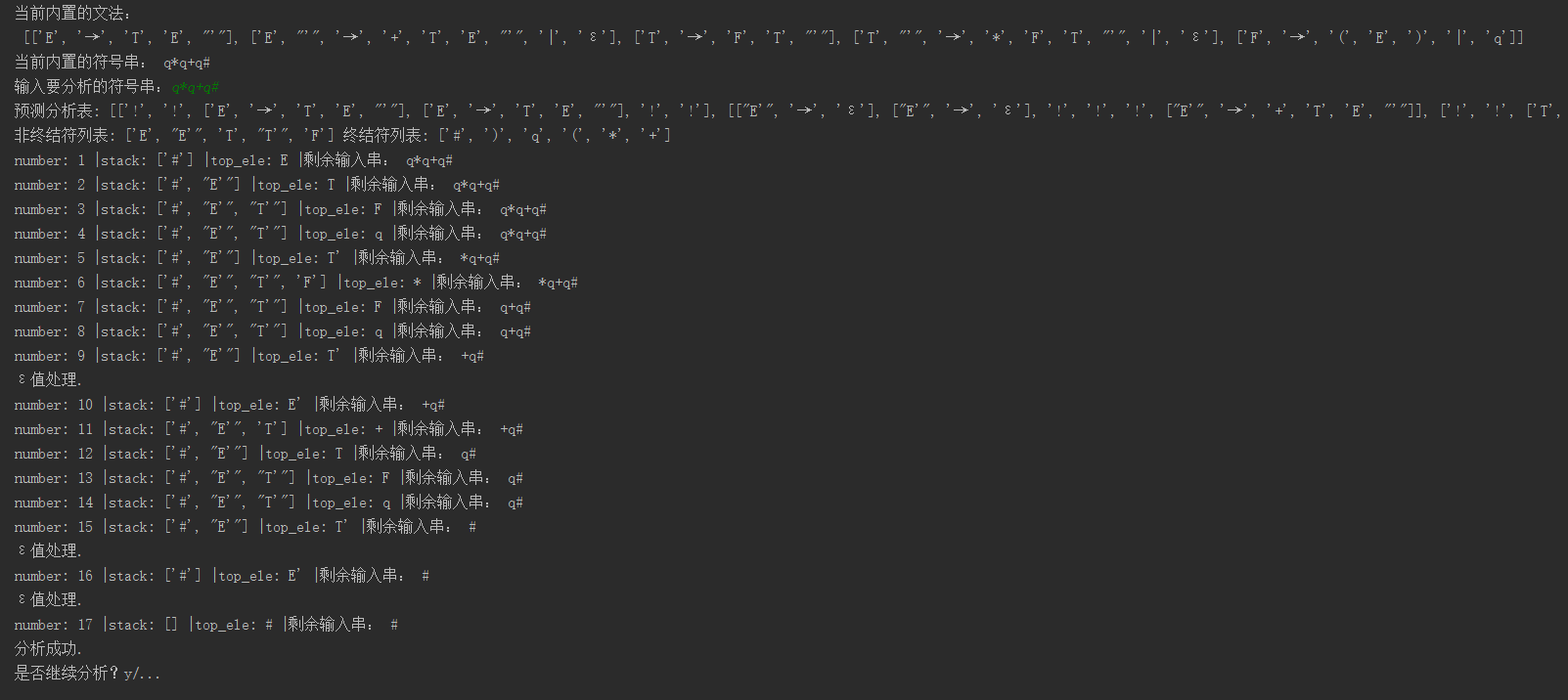# 总结

• 同步更新至CSDN，仅作实验记录之用。
• 水平有限，文章有需要改正之处还望指出。
展开全文• 国内疫情统计及预测分析系统

系统目的

采集国外肺炎疫情的数据，通过echarts以图表的方式展示

软件架构说明

1、数据采集/爬虫模块

该模块的功能是定时采集全球最新的疫情数据

采集的目标网站为丁香园，目标地址为https://3g.dxy.cn/newh5/view/pneumonia?scene=2&clicktime=1579582238&enterid=1579582238&from=timeline&isappinstalled=0l;

涉及的技术有使用requests采集网页信息、使用re正则表达式提取网页中的数据、使用json解析数据、使用pymysql连接数据库、使用apscheduler启动定时任务定时

采集每天最新的疫情数据

2、数据展示/web可视化模块

该模块的主要功能是根据采集到的的数据进行可视化分析展示，从不同的角度分析疫情的走向，了解疫情的最新动态

mysql数据统计分析的sql语句的编写以及使用线性回归预测分析未来七天的疫情数据

系统演示视频：国内新冠疫情分析系统.zip - 蓝奏云

系统截图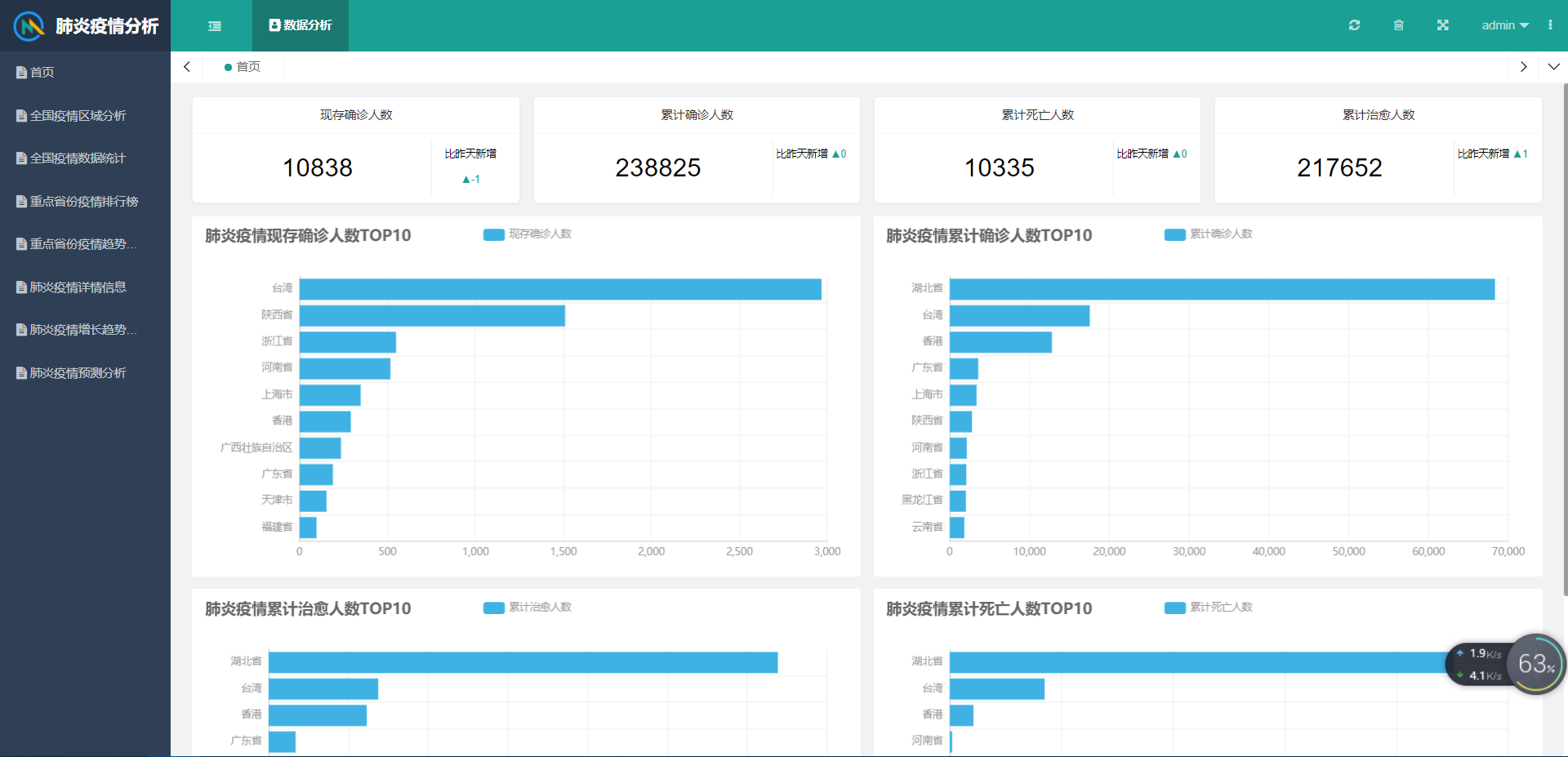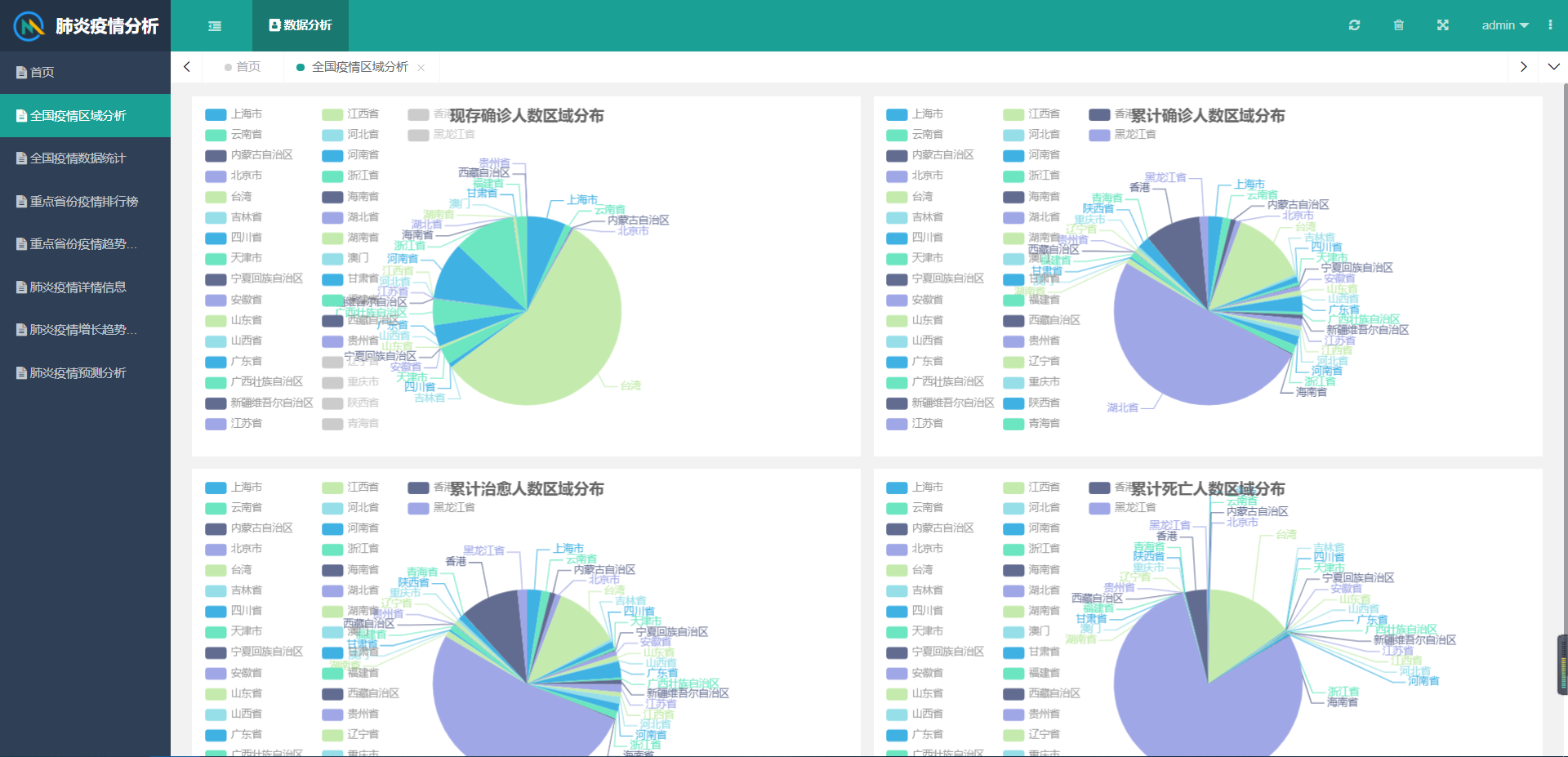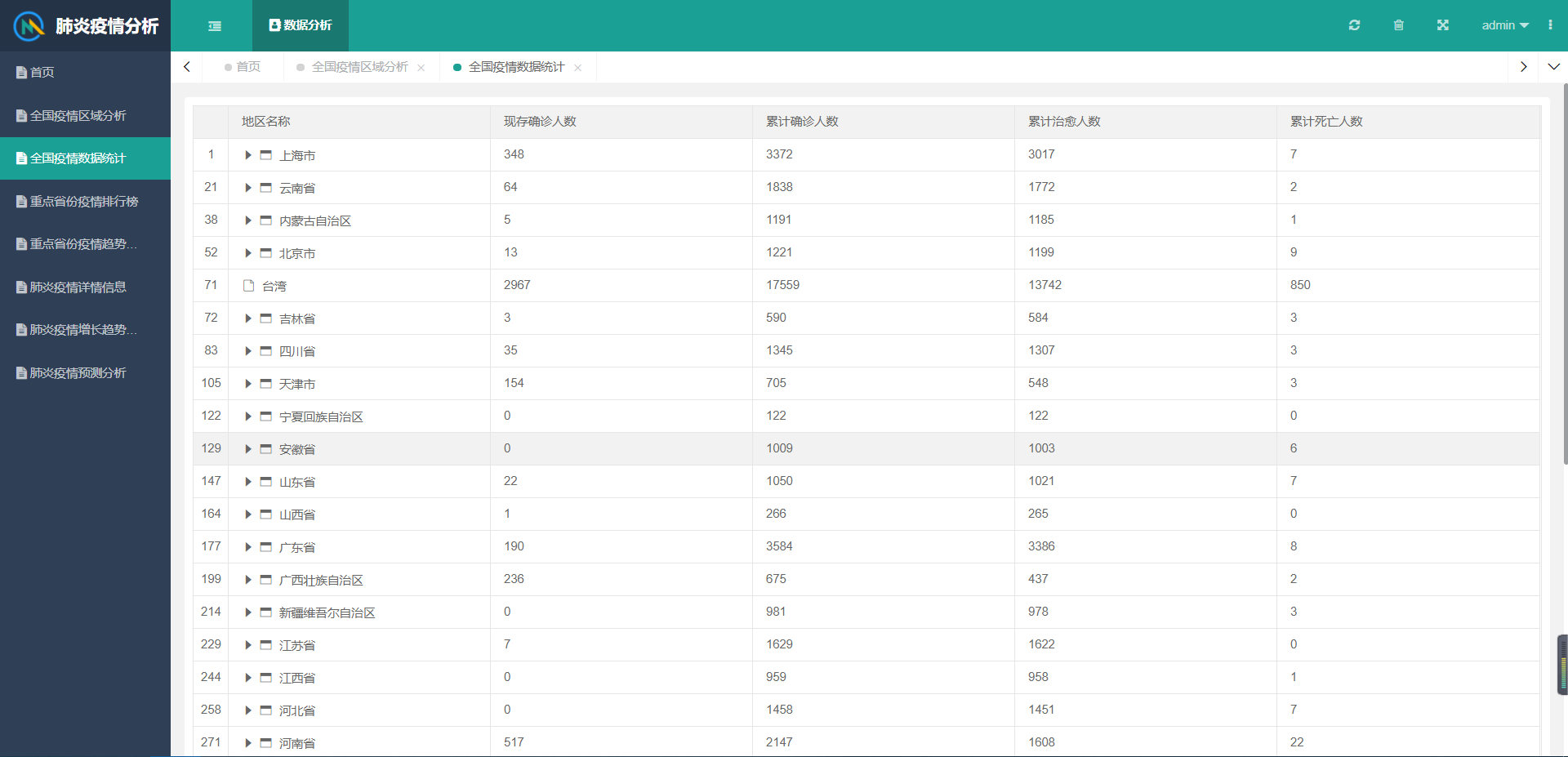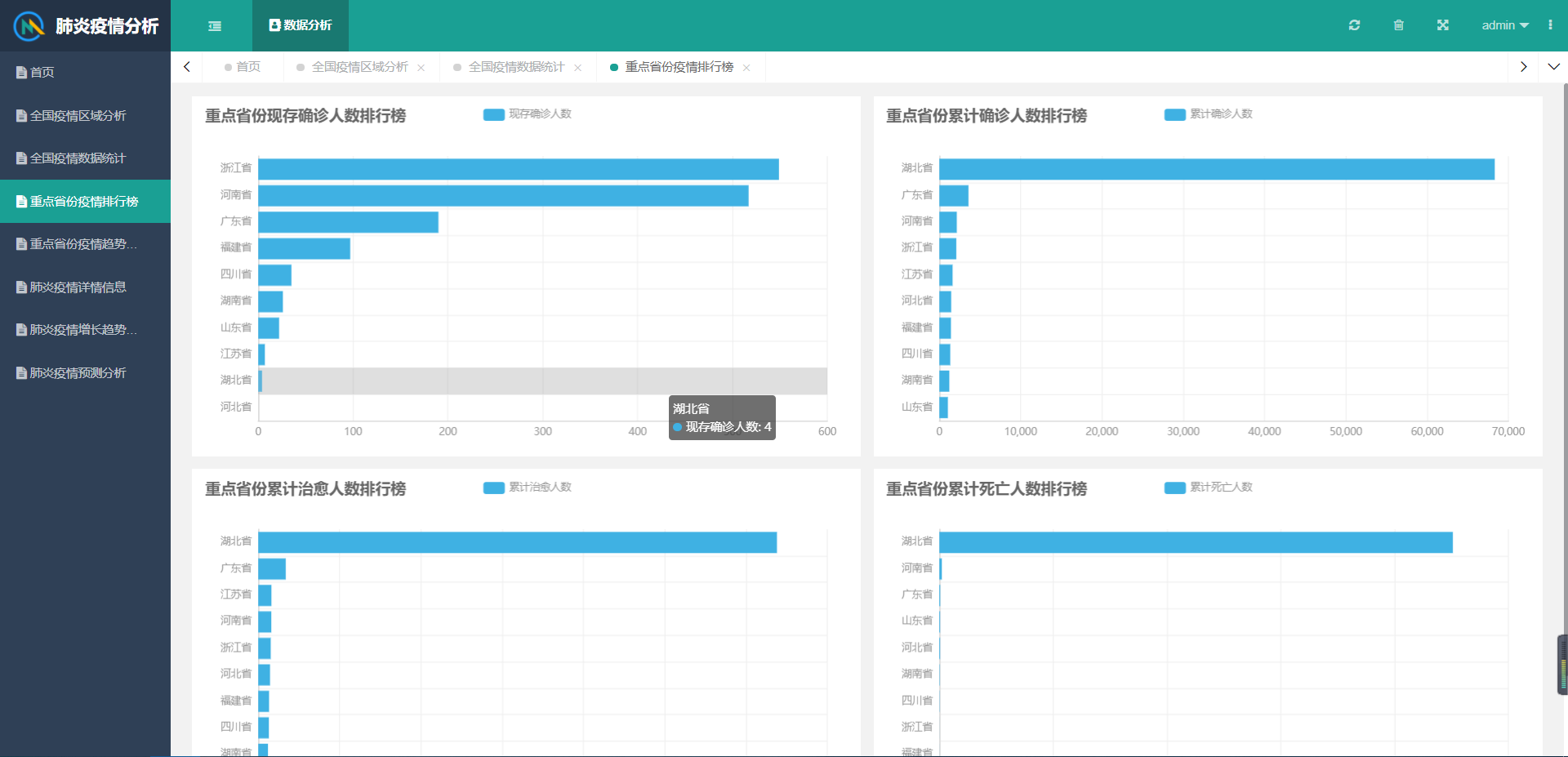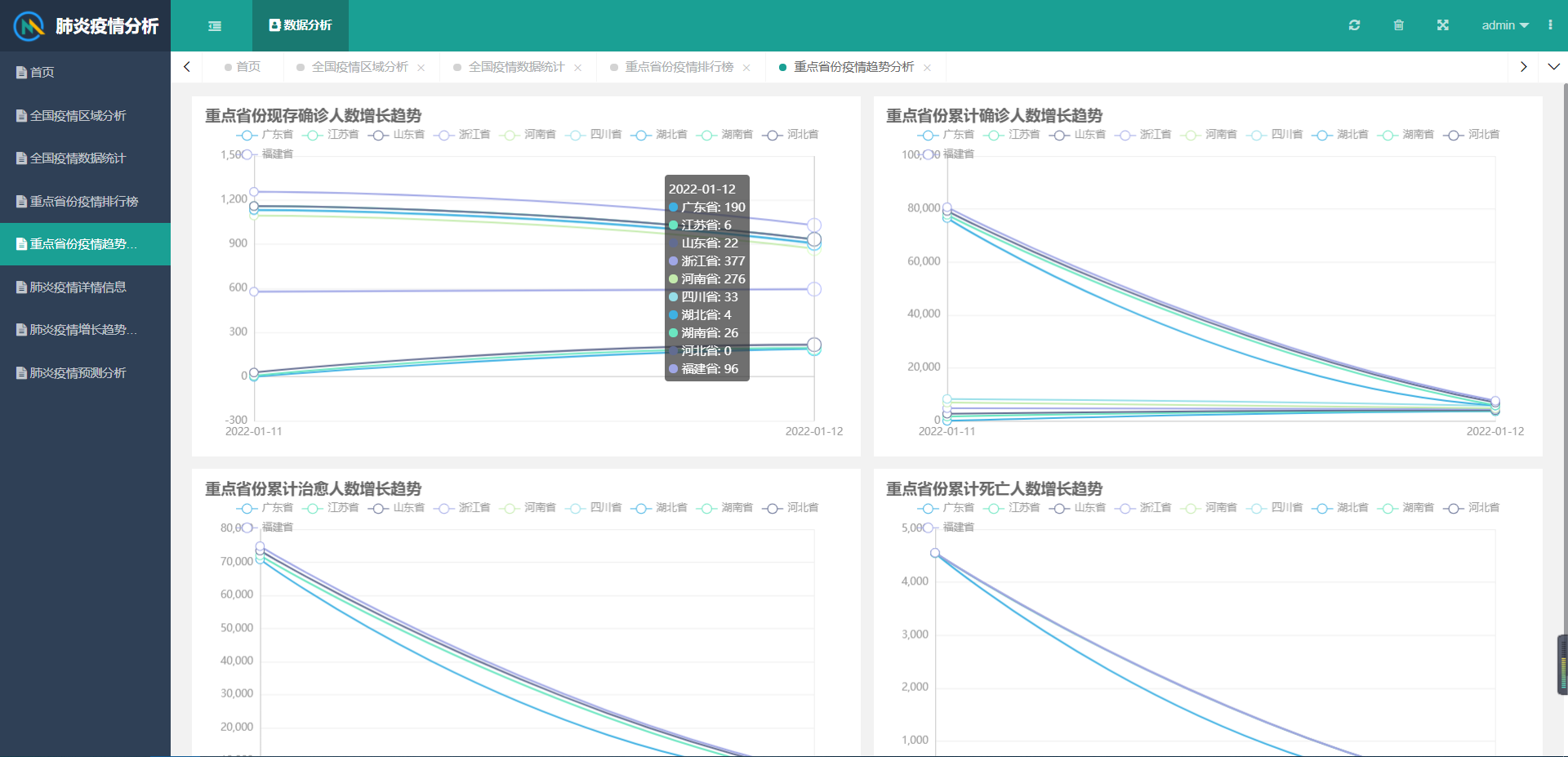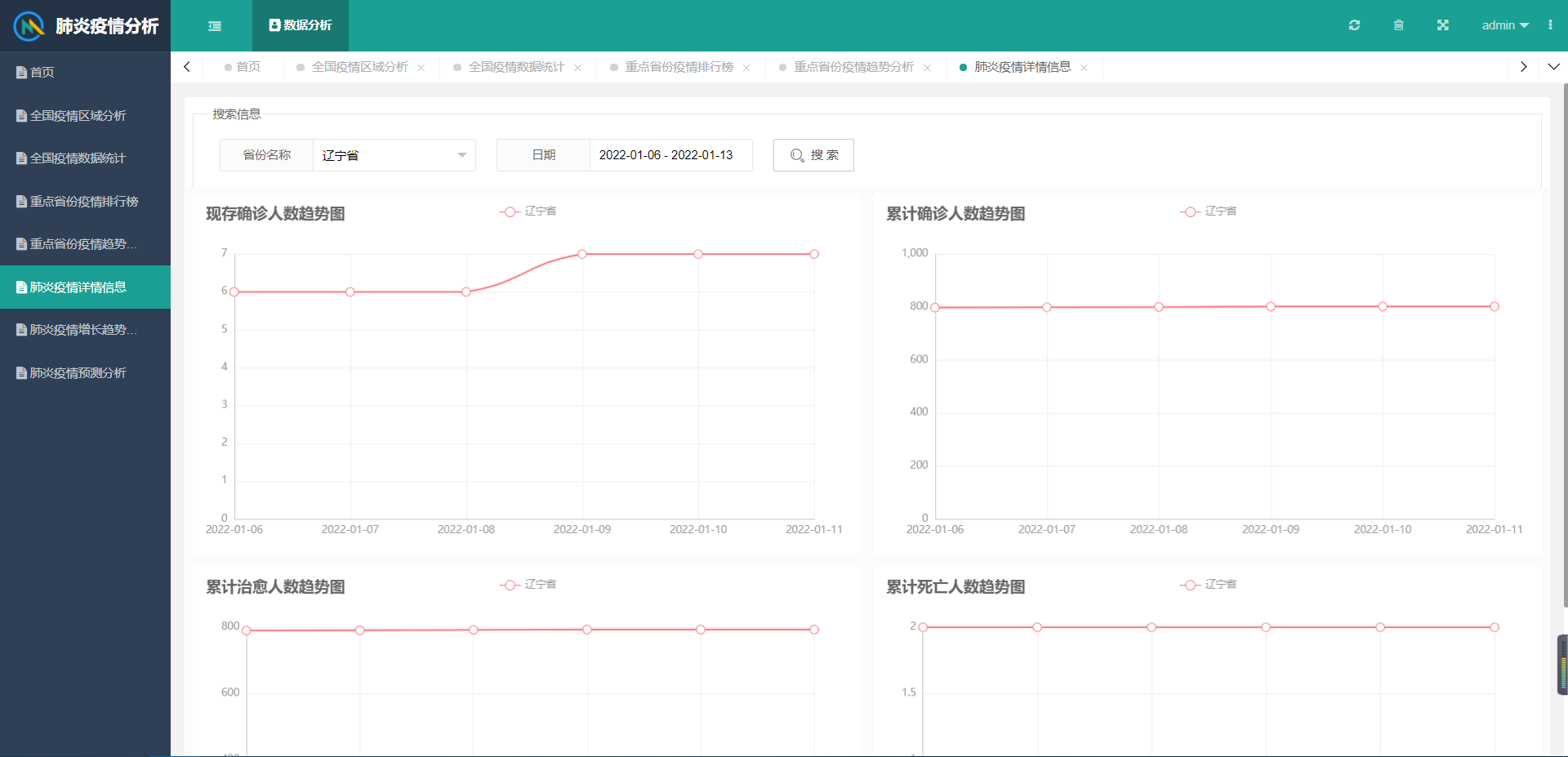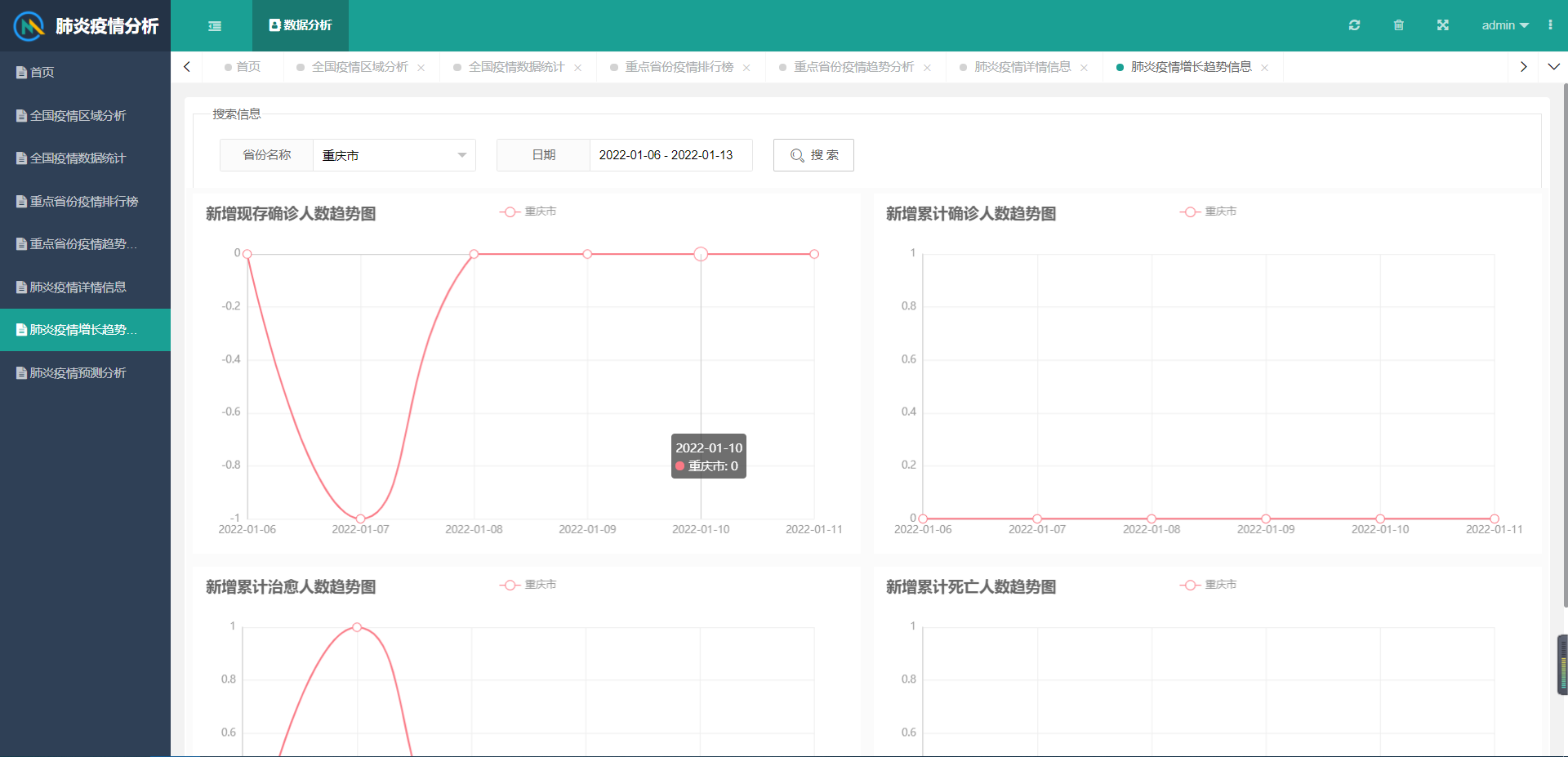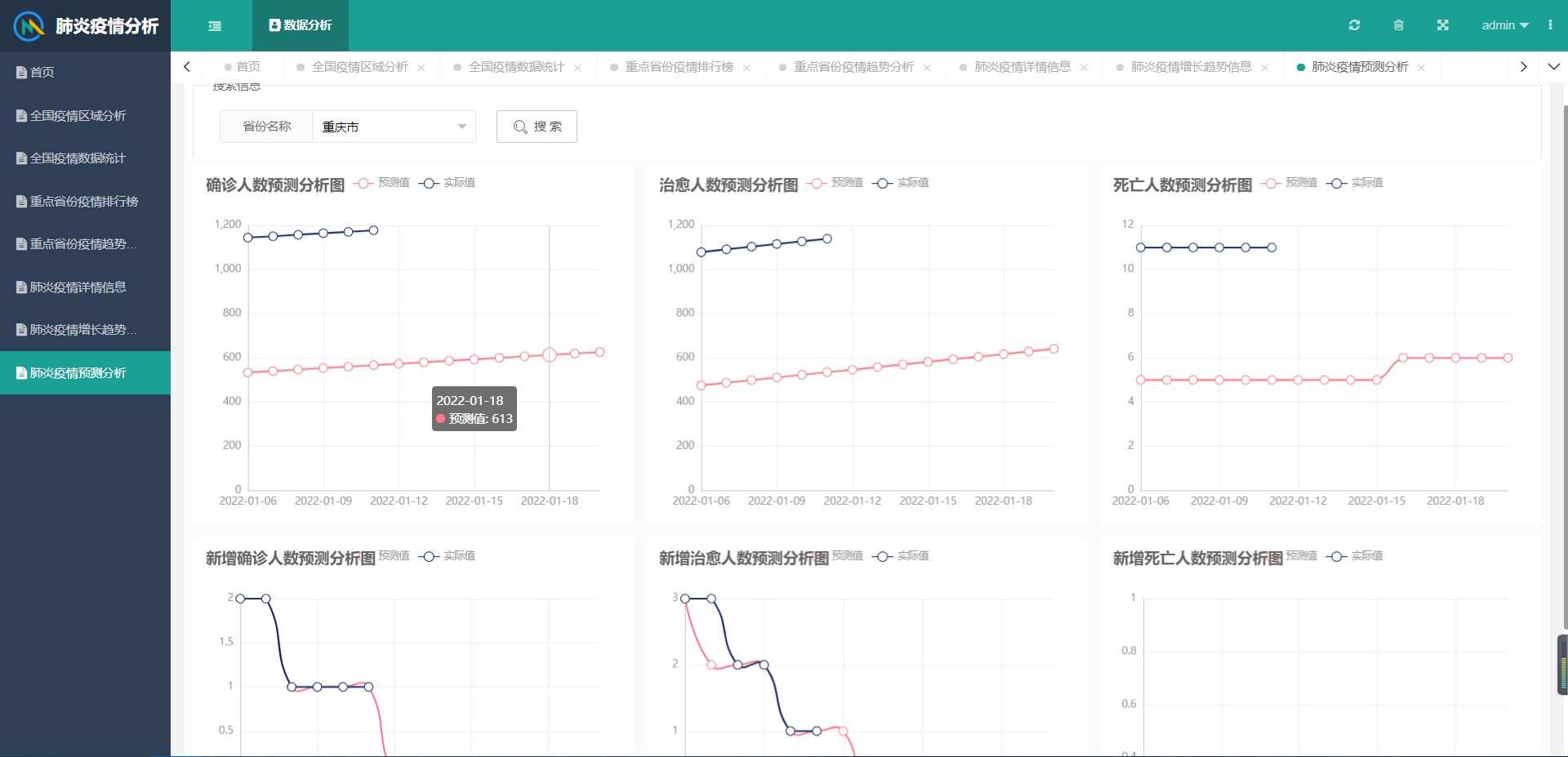展开全文python flask 前端
• Python数据分析-房价的影响因素图解 上一篇OF讲述了房价的影响因素，主要是房屋面积、卫生间数、卧室数。今天，我们通过建立模型来预测房价。机器学习中关于回归算法-数据发展的预测，包含了几个模型： 1、线性...python sklearn 机器学习
• 实验2《预测分析法设计与实现》 一、实验目的   加深对语法分析器工作过程的理解；加强对预测分析法实现语法分析程序的掌握；能够采用一种编程语言实现简单的语法分析程序；能够使用自己编写的分析程序对简单的...
• 摘要：大数据分析要实现的应用领域之一就是预测分析，可视化分析和数据挖掘都是前期铺垫工作，只要在大数据中挖掘出信息的特点与联系，就可以建立科学的数据模型，通过模型带入新的数据，从而预测未来的数据。...大数据 数据挖掘
• 目录相关链接1 题目2 Python实现的baseline2.1 数据读取2.2 温度特征处理2.3 天气状况特征处理2.4 风向特征处理2.5 天气进行有序...电力系统负荷（电力需求量，即有功功率）预测是指充分考虑历史的系统负荷、经济状况、
• 预测分析程序的实现 设计内容及要求： 对文法 G: E-&gt;TE' E'-&gt;+TE' E'-&gt;e T-&gt;FT' T'-&gt;*FT' T'-&gt;e F-&gt;(E) F-&gt;i  造出 G 的表驱动的预测分析程序...
• 课程编号：400802020 课程名称：数据分析预测课程设计 ...运用所学的机器学习知识，基于Python或R或C编程环境，根据下面给出的要求，选择相关分析设计内容，完成一个数据分析预测案例实现，最终提交设计文档...
• 1.实验内容1、定义一个LL(1)文法，示例如(仅供参考) G[E]：E →TE' E'→+TE'|εT →FT' T' → *FT'|εF → i|(E) 2、构造其预测分析表，如3、LL(1)文法的预测分析表的模型示意图 4、运行结果，示例如下2....编译原理实验 python
• 为给定文法写预测分析程序，预测分析表已知（虽然求取预测分析表更重要，但是老师要求是给定预测分析表的情况下写预测分析程序），文法如下： E–>TE’ E’–> +TE’|ε T–>FT’ T’–> *FT’|ε F–>(E...编译原理 c++
• 日理解预测分析程序的构造过程。 ④能够使用某种高级语言实现一个预测分析程序。 实验内容 编写一个预测分析程序，能实现以下功能： ①给定文法G，消除文法G的左递归和左公因子； ②构造并输出各非终结符的FIRST集和...编译原理 c语言
• 前面学习了Excel中的相关分析，在数据分析中，相关分析和回归分析关系...　我们在得到两组数据之间的相关程度之后，就可以使用回归分析进行预测了，换言之，相关分析是回归分析的基础和前提，回归分析是相关分析的深...逻辑回归 数据挖掘 机器学习
• 但不可能每次都那么费劲的自己人工去看盘分析, 所以结合所学,就有这个项目. foot-parent 是一个集足球数据采集器,简单分析,同步到微信及其他发布平台一体化的项目. 程序采用go语言开发,项目结构清晰完整,非常容易...go 人工智能 大数据
• 此项目还未写完 一 项目背景介绍 基于新闻咨询行业的头条数据进行的准实时处理的数据仓库...内容数据 目的： 构建数仓模型，分析这些数据的价值 两种分析模型： 用户行为分析模型： 事件分析，留存分析，漏斗分析 D数据仓库
• 实验二 预测分析算法的设计与实现 （8学时） 一、实验目的 通过预测分析算法的设计与实现，加深对自上而下语法分析方法的理解，尤其是对自上而下分析条件的理解。 二、实验要求 输入文法及待分析的输入串，输出...编译原理
• 项目为《Python 数据分析与挖掘实战》第 13 章：财政收入影响因素分析预测模型。项目实现了因变量的筛选，阐述了灰色预测原理计算过程，实现了灰色预测和神经网络的结合模型。神经网络
• ## 二手车价预测分析

千次阅读 多人点赞 2019-10-11 22:02:05
数据分析：二手车价格预测分析 目录 一、项目要求 根据二手车市场交易相关数据，分析二手车价格的影响因素，并创建预测模型，预测二手车交易价格。 二、数据预处理 导入模块 import pandas as pd import numpy as ...
• 学习内容： 提示：这里可以添加要学的内容 例如： 1、 搭建 Java 开发环境 2、 掌握 Java 基本语法 3、 掌握条件语句 4、 掌握循环语句 学习时间： 提示：这里可以添加计划学习的时间 例如： 1、 周一至周五晚上 7 ...神经网络 机器学习 算法
• 电信用户流失分析预测一、项目背景二、分析目的三、数据来源四、提出问题五、分析流程六、理解数据1.特征理解2.导入数据3.数据转化七、用户流失分析1.流失占比分析2.用户属性分析3.用户属性分析4.合同属性分析八、...python 机器学习
• 在我国现行的分税制财政管理体制下，地方财政收人不仅是国家财政收入的重要组成部分，而且具有其相对独立的构成内容。如何有效的利用地方财政收入，合理的分配，来促进地方的发展，提高市民的收入和生活质量是每个...数据挖掘
• 本篇主要从两方面去介绍传统的时间序列分析方法，一是时间序列数据的统计描述，二是其预测方法。
• 2022年商用密码行业市场规模前景调研分析预测及投资建议可行性研究预测 （1）中国商用密码行业发展情况：中国商用密码发展起步较晚，历程始于近现代密码时代。整体来看，我国商用密码经历了起步形成、快速发展、...数据仓库
• 数据分析预测是一项重要的内容，其中也将使用四维分析。但是也一定要了解数据分析预测用哪一些常见的方法。 　1、描述型分析 　这是数据分析预测过程中比较常见。在业务中，这种方法向数据分析师提供了非常...
• 针对特定的预测问题，只是拥有数据还不够，想要从纷繁复杂的数据关系中挖掘出可用于预测的规律或模式，还得运用恰当的分析方法。比如聚类分析，恰当地选择聚类算法，可以按维度将数据...本内容将分别介绍常用的分析...
• 实验 预测分析表方法 一、实验目的 理解预测分析表方法的实现原理。 二、实验内容 编写一通用的预测法分析程序，要求有一定的错误处理能力，出错后能够使程序继续运行下去，直到分析过程结束。可通过不同的文法...
• 01 引言 近些年，智能制造在流程工业生产中得到了示范应用，其重要性逐渐深入人心。国内外学者对于智能制造的理解和定义不尽相同。...预测性维护是以状态为依据的维修，是对设备进行连续在线的状态监测及数据分析设备管理系统
• 《统计预测与决策》第五版_徐国祥 文章目录一、干预分析模型二、干预分析模型的基本形式（1）干预变量形式（2）干预事件形式三、单变量干预分析模型的识别与估计（1）干预模型的构造（2）干预效应的识别四、干预模型...
• 本文详述了如何通过数据预览，基本数据分析、探索式数据分析，缺失数据填补等方法，实现对Kaggle上根据比特币历史数据集预测未来走势的问题进行数据分析的探索式实践。 数据来源：Kaggle—Bitcoin Historical Data机器学习...